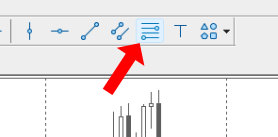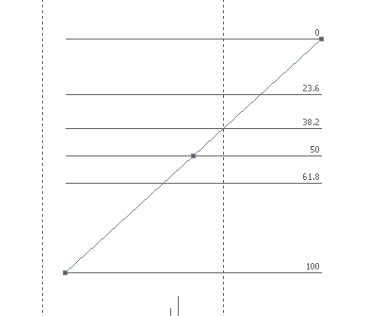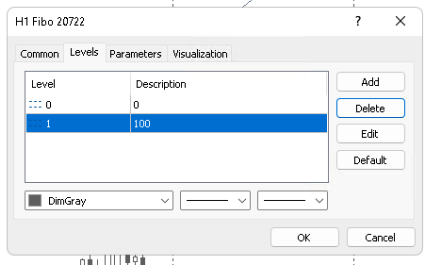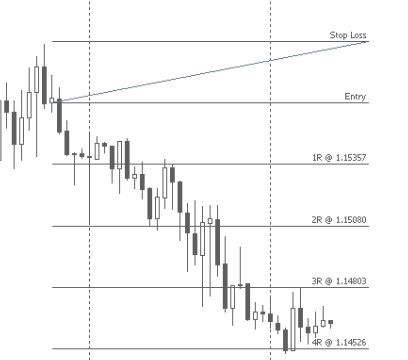You don't need to buy a separate indicator or EA to see the risk/reward ratio on every trade. MT5 already has a built-in measurement tool to do that.

The Fibonacci Retracement tool in MT5 can be easily altered to show the risk/reward ratio on any trade. Open the settings of the tool, then add risk multiples to the Levels tab.

Once you know this trick, you'll probably find yourself using this tool a lot.

Here's how to do it…

## Tutorial Video

?

Watch more of my videos on my YouTube channel.

## Risk/Reward Ratio Calculation

This ratio is commonly known as the risk/reward ratio, but it should be more accurately referred to as the reward/risk ratio.

The ratio is actually calculated with the amount of reward on the top of the fraction and the risk on the bottom.

For example, if a closed trade has 500 pips of profit and had 100 pips of risk, the formula looks like this:

500 / 100 = 5

So the reward/risk ratio in this example is 5.

That's how the reward to risk ratio will be shown in the MetaTrader 5 tool.

## Why Reward/Risk is Important in Trading

For example, if you have an average reward to risk ratio of 1 on your trades, this means that you should have a greater than 50% win rate in order to be profitable.

If you have a lower win rate, your average reward/risk will have to be higher to be profitable.

Then of course, you don't need to have a high reward/risk ratio if you have a high win rate.

Now that you understand how it works, let's get into how to add it to your MetaTrader 5 chart.

## Add the Fibonacci Tool to a Chart

This is what it looks like on your toolbar.Click anywhere on the chart, then drag the tool, so the tool expands and you can see the levels.

At first, you'll see the traditional Fibonacci Retracement tool settings.If you're going to use the tool to measure Fibonacci retracements, then keep these settings.

Otherwise, you can delete the retracement settings in the next step.

## Customize the Tool

Now that you have the tool on your chart, first make sure that the squares are displayed on the diagonal line.

You'll see the squares in the screenshot below.

If the squares aren't there, then double click on the diagonal line to show the squares.

Once you see the squares, right-click on the diagonal line to show the Fibo settings menu.Then select Properties…

First, delete the unnecessary levels.Then add the first level.

Risk will be determined by the distance between the 0 and 1 levels.

Therefore, in order to create a 1 times risk measurement, we have to create a level that has the same size as the distance between 0 and 1.

So the first level will be 2 because that's 1 larger than 1.  You can put anything you want into the description.

I use 1R, or 1 times risk.Add as many levels as you want.

I like to go up to 10R.

Here's what it looks like on the chart.

This move had a potential 7.5R of profit.You can also create fractions of a level, if that's something you use in your trading.

For example, you could set a 1.5R level.

## How to Use the Reward/Risk Tool

Once you've customized the Fibonacci tool and turned it into a reward/risk tool, the settings will stick until you change the settings again.

Now that you have this set up, let's take a look at how to actually use it.

There are a couple of ways that you can use this tool…

First, it can be used to check if there's enough room for you to make the risk multiple that you want to make on a trade you're about to take.

For example, if your strategy requires a minimum 1R profit target, you can easily see where price would have to get to on your chart, in order for you to make 1R.

If that profit target seems reasonable given the current price action, then you can take the trade. Otherwise, you can pass.

Next, you can evaluate closed trades to see how much profit you could have made.

If enough of your trades have more potential profit than actual profit, then you could look for ways to trail your stop to make more profit.

## Bonus: Add the Price to Each Reward/Risk Line

Now you can see your reward levels on your MT5 chart, but wouldn't it be great to see the actual price that the levels are at?

Luckily, there's an easy way to do this in MT5.

On each level, add the following to the description of each line to show the price that the line is at.

%\$

While you're at it, you can also change the 0 and 100 levels to Stop Loss and Entry, respectively.

This is what my settings look like.This is what it looks like on the chart.After you select the tool, start by clicking on the entry price of your trade.

Then drag the tool to your stop loss price.

Now you can see the exact price of all the profit levels.

This makes it easy to enter take profit levels when you're trading.

## Final Thoughts

This simple hack to the Fibonacci Retracement tool creates a free and easy way to measure risk vs reward.

Set it up once and you'll always have it handy.

You can also use this method on other trading platforms, when you want an easy to use reward/risk tool.

Get more MetaTrader 5 tutorials here.